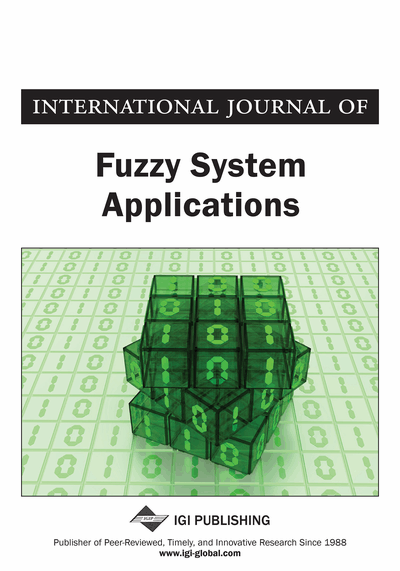# Semidefinite Programming-Based Method for Implementing Linear Fitting to Interval-Valued Data

Minghuang Li, Fusheng Yu
DOI: 10.4018/ijfsa.2011070103
OnDemand:
(Individual Articles)
Available
\$37.50
No Current Special Offers

## Abstract

Building a linear fitting model for a given interval-valued data set is challenging since the minimization of the residue function leads to a huge combinatorial problem. To overcome such a difficulty, this article proposes a new semidefinite programming-based method for implementing linear fitting to interval-valued data. First, the fitting model is cast to a problem of quadratically constrained quadratic programming (QCQP), and then two formulae are derived to develop the lower bound on the optimal value of the nonconvex QCQP by semidefinite relaxation and Lagrangian relaxation. In many cases, this method can solve the fitting problem by giving the exact optimal solution. Even though the lower bound is not the optimal value, it is still a good approximation of the global optimal solution. Experimental studies on different fitting problems of different scales demonstrate the good performance and stability of our method. Furthermore, the proposed method performs very well in solving relatively large-scale interval-fitting problems.
Article Preview
Top

## Introduction

The objective of linear regression is to adjust the parameters of a linear model function so as to best fit a given data set. In the real world, the observations and measurements are usually subject to imprecision and vagueness. In order to assess such uncertainty one ideal way is to represent the data as intervals or fuzzy sets.

The linear regression analysis involving fuzzy data is the central concern of fuzzy regression. Tanaka (1982) first proposed a fuzzy linear regression model by minimizing the index of fuzziness of the system. Diamond (1990, 1994, 1997) introduced a metric on the set of fuzzy numbers and used this metric to define a least-sum-of-squares criterion function as in the usual sense. Further discussion can be found in (Chang, 2001; Coppi et al., 2004; D’Urso, 2003, 2006; Tanaka et al., 1982, 1987, 1996; Sakawa et al., 1992; Ma et al., 1997; Chang et al., 2001; Wu, 2003) and other references therein.

At the interval level, the similar topic has also been discussed extensively in the field of symbolic data analysis (Bock et al., 2000). Bertrand and Goupil (2000) and Billard and Diday (2000, 2003) introduced statistics methods based on covariance and correlation function suitable for interval-valued data. A centre and range method was developed in Lima Neto et al. (2008) for fitting interval data. Various forms of support vector interval regression approaches have been discussed (An et al., 2008; Jeng et al., 2003; Hong et al., 2005, 2006). Additionally, in practical context, this model has shown its potential in forecasting interval-valued time series (Maia et al., 2008).

The model studied in this article is fitting a crisp linear function to interval-valued input-output data. More precisely, the model consists of minimizing the sum of squared interval distances between observed and predicted values. The predicted values are calculated by interval arithmetic (or the extension principle).

## Complete Article List

Search this Journal:
Reset
Volume 13: 1 Issue (2024)
Volume 12: 1 Issue (2023)
Volume 11: 4 Issues (2022)
Volume 10: 4 Issues (2021)
Volume 9: 4 Issues (2020)
Volume 8: 4 Issues (2019)
Volume 7: 4 Issues (2018)
Volume 6: 4 Issues (2017)
Volume 5: 4 Issues (2016)
Volume 4: 4 Issues (2015)
Volume 3: 4 Issues (2013)
Volume 2: 4 Issues (2012)
Volume 1: 4 Issues (2011)
View Complete Journal Contents Listing